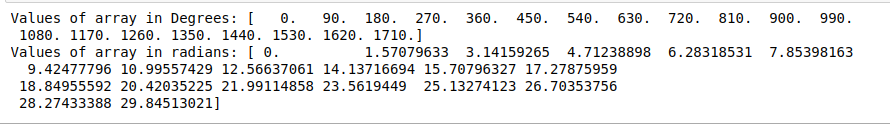Convert angles from degrees to radians for all elements in a given NumPy array

• Last Updated : 29 Aug, 2020

This method takes an array as an input parameter and returns an array that has radian values.

Python

Output:This method takes input array and returns an array that has radian values the same as the size of the input array.

Python3

Output:Method 3: Using Formula

Python3

 # python code demonstrating usage of radians# method to convert degrees to radians# importing numpy libraryimport numpy as npimport math  # initialising an arrayarray=np.arange(20.)*90  # printing degree valuesprint('Values of array in Degrees:',array)radian_array=[]  # converting to radiansfor i in array:    radian_array.append(i*math.pi/180)  # printing radian valuesprint('Values of array in radians:',radian_array)

Output:My Personal Notes arrow_drop_up